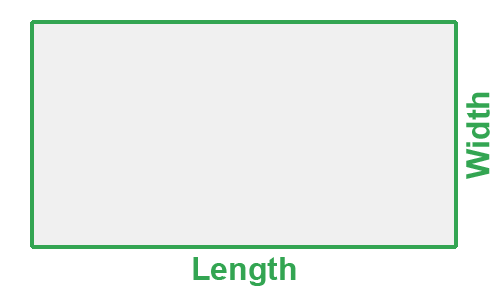# Square Footage Calculatorft
in
ft
in

X

Use our square footage calculator to calculate the area in square feet, square yardage, square inch, acre or section.

## Definition of a square calculator

A square footage calculator is an automated program used to calculate the number of square feet of a given area. Square footage is calculated by multiplying width by length or width by height. Typically in the US, feet, and inches are used for measuring.

## How to calculate square footage?

1. Determine the width and length. Length can also be height.
2. If your width and length are not measured in feet convert measurements into feet.
3. For inches divide by 12
4. For yards multiply by 3
5. For centimeters multiply by 0.03281
6. For meters multiply by 3.281
7. Finally, multiply Length (ft) x Width (ft) = Square Footage

## Square footage equation

L x W = A
W = width, L = length, A = area

## Examples using square footage

Mr. Calculate needs to tile his bathroom but needs to find the square footage. His bathroom is 5' wide and 8' long.

5 x 8 = 3 ft2

Mr. Calculate loved his newly tiled bathroom so much he decided to tile the kitchen floor. His kitchen floor is 12 feet and 3 inches wide and 12 feet and 9 inches long.

12 + (3 / 12) = 12.25

12 + (9 / 12) = 12.75

12.25 x 12.75 = 156.1875 ft2

## Popular square footage areas

Disclaimer: All calculators, tools, and results featured on calculateconvert.com are to be finalized and not be considered a quotation or binding contract.
Proofs and formulas are provided as references.